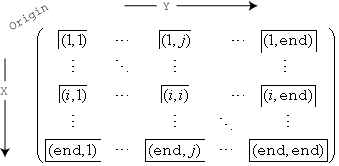# histcounts2

## 语法

``[N,Xedges,Yedges] = histcounts2(X,Y)``
``[N,Xedges,Yedges] = histcounts2(X,Y,nbins)``
``[N,Xedges,Yedges] = histcounts2(X,Y,Xedges,Yedges)``
``[N,Xedges,Yedges] = histcounts2(___,Name,Value)``
``[N,Xedges,Yedges,binX,binY] = histcounts2(___)``

## 说明

``[N,Xedges,Yedges] = histcounts2(X,Y)` 将 `X` 和 `Y` 中的值划分为多个二维 bin，并返回 bin 计数以及每个维度的 bin 边界。`histcounts2` 函数使用自动分 bin 算法，然后返回均匀 bin，这些 bin 可涵盖 `X` 和 `Y` 中值的范围并显示分布的基本形状。`

``[N,Xedges,Yedges] = histcounts2(X,Y,nbins)` 指定要在每个维度中使用的 bin 数量。`

``[N,Xedges,Yedges] = histcounts2(X,Y,Xedges,Yedges)` 按照 `Xedges` 和 `Yedges` 指定的 bin 边界，将 `X` 和 `Y` 划分为多个 bin。`N(i,j)` 对符合 `Xedges(i)` ≤ `X(k)` < `Xedges(i+1)` 且 `Yedges(j)` ≤ `Y(k)` < `Yedges(j+1)` 条件的值 `[X(k),Y(k)]` 进行计数。每个维度中的最后 bin 还包含最后一个（外部）边界。例如，如果 `Xedges(end-1)` ≤ `X(k)` ≤ `Xedges(end)` 且 `Yedges(i)` ≤ `Y(k)` < `Yedges(i+1)`，则 `[X(k),Y(k)]` 属于最后一行中的第 `i` 个 bin。`

``[N,Xedges,Yedges] = histcounts2(___,Name,Value)` 还可使用一个或多个 `Name,Value` 对组参数（采用上述语法中有任意输入参数）指定的其他选项。例如，可以指定 `'BinWidth'` 和一个二元素向量来调整每个维度中 bin 的宽度。`

``[N,Xedges,Yedges,binX,binY] = histcounts2(___)` 还使用任何上述语法返回索引数组 `binX` 和 `binY`。`binX` 和 `binY` 数组的大小与 `X` 和 `Y` 相同，其元素是 `X` 和 `Y` 中对应元素的 bin 索引。第 `(i,j)` 个 bin 中的元素数量等于 `nnz(binX==i & binY==j)`；若 `Normalization` 为 `'count'`，则它与 `N(i,j)` 相同。`

## 示例

```x = randn(100,1); y = randn(100,1); [N,Xedges,Yedges] = histcounts2(x,y)```
```N = 7×6 0 0 0 2 0 0 1 2 10 4 0 0 1 4 9 9 5 0 1 4 10 11 5 1 1 4 6 3 1 1 0 0 1 2 0 0 0 0 1 0 1 0 ```
```Xedges = 1×8 -3 -2 -1 0 1 2 3 4 ```
```Yedges = 1×7 -3 -2 -1 0 1 2 3 ```

```x = [1 1 2 3 2 2 1 1 2 3]; y = [5 6 3 8 9 1 2 7 5 1]; nbins = [3 4]; [N,Xedges,Yedges] = histcounts2(x,y,nbins)```
```N = 3×4 1 0 2 1 1 1 1 1 1 0 0 1 ```
```Xedges = 1×4 0.6000 1.4000 2.2000 3.0000 ```
```Yedges = 1×5 0 2.3000 4.6000 6.9000 9.2000 ```

```x = randn(1000,1); y = randn(1000,1); Xedges = -5:5; Yedges = [-5 -4 -2 -1 -0.5 0 0.5 1 2 4 5]; N = histcounts2(x,y,Xedges,Yedges)```
```N = 10×10 0 0 0 0 0 0 0 0 0 0 0 0 0 0 1 1 1 0 0 0 0 0 5 5 3 5 1 2 0 0 0 2 19 23 29 25 26 20 5 0 0 10 36 51 59 71 54 46 10 0 0 7 43 46 79 64 60 46 9 0 0 3 12 18 21 23 19 9 6 0 0 0 5 3 2 8 2 2 0 0 0 0 0 1 1 1 0 0 0 0 0 0 0 0 0 0 0 0 0 0 ```

```x = randn(1000,1); y = randn(1000,1); [N,Xedges,Yedges] = histcounts2(x,y,6,'Normalization','probability')```
```N = 6×6 0 0 0.0020 0.0020 0 0 0 0.0110 0.0320 0.0260 0.0070 0.0010 0.0010 0.0260 0.1410 0.1750 0.0430 0.0060 0 0.0360 0.1620 0.1940 0.0370 0.0040 0 0.0040 0.0300 0.0370 0.0100 0.0010 0 0.0030 0.0040 0.0040 0.0010 0 ```
```Xedges = 1×7 -4.0000 -2.7000 -1.4000 -0.1000 1.2000 2.5000 3.8000 ```
```Yedges = 1×7 -4.0000 -2.7000 -1.4000 -0.1000 1.2000 2.5000 3.8000 ```

```x = randi([-10,10],1000,1); y = randi([-10,10],1000,1); [N,Xedges,Yedges,binX,binY] = histcounts2(x,y,'BinMethod','integers');```

`[x(3),y(3)]`
```ans = 1×2 -8 10 ```
`bin = [binX(3) binY(3)]`
```bin = 1×2 3 21 ```

## 输入参数

`X``Y` 中的对应元素指定二维数据点 `[X(k),Y(k)]` 的 x 和 y 坐标。`X``Y` 的数据类型可以不同。

`histcounts2` 忽略所有的 `NaN` 值。同样，`histcounts2` 忽略 `Inf``-Inf` 值，除非 bin 边界将 `Inf``-Inf` 显式指定为 bin 边界。

• 如果 `nbins` 是标量，则 `histcounts2` 在每个维度中使用该标量所指定的 bin 数。

• 如果 `nbins` 是向量，则 `nbins(1)` 指定 x 维度的 bin 数，`nbins(2)` 指定 y 维度的 bin 数。

x 维度中的 bin 边界，指定为向量。`Xedges(1)` 是 x 维度的第一个 bin 的第一个边界，`Xedges(end)` 是最后一个 bin 的外边界。

y 维度中的 bin 边界，指定为向量。`Yedges(1)` 是 y 维度的第一个 bin 的第一个边界，`Yedges(end)` 是最后一个 bin 的外边界。

### 名称-值参数

`'auto'`

`'scott'`

`'fd'`

Freedman-Diaconis 规则对数据中的离群值不太敏感，可能更适用于重尾分布的数据。它使用 bin 大小 `[2*IQR(X(:))*numel(X)^(-1/4), 2*IQR(Y(:))*numel(Y)^(-1/4)]`，其中 `IQR` 为四分位差。

`'integers'`

`histcounts2` 并不始终使用这些精确的公式来选择 bin 数目。有时 bin 数目会稍做调整，以便 bin 边界落在“合适”的数字上。

x 维度的 bin 范围，指定为二元素向量 `[xbmin,xbmax]`。该向量表示 x 维度中的第一个和最后一个 bin 边界。

y 维度的 bin 范围，指定为二元素向量 `[ybmin,ybmax]`。该向量表示 y 维度中的第一个和最后一个 bin 边界。

• ${v}_{i}$ 是 bin 的值。

• ${c}_{i}$ 是 bin 中的元素数目。

• ${A}_{i}={w}_{xi}\cdot {w}_{yi}$ 是每个 bin 的面积，使用 bin 宽度 x 和 y 计算得出。

• $N$ 是输入数据中的元素数目。此值可以大于分 bin 数据元素数，前提是数据中包含 `NaN` 值，或者某些数据位于 bin 范围外。

bin 值注释
`'count'`（默认值）

`${v}_{i}={c}_{i}$`

• 观测值的计数或频率。

• bin 值之和小于或等于 `numel(X)``numel(y)`。仅当某些输入数据不在 bin 中时，bin 值之和才会小于 `numel(X)`

`'countdensity'`

`${v}_{i}=\frac{{c}_{i}}{{A}_{i}}$`

• 按 bin 的面积进行换算的计数或频率。

• bin 的体积（N 值 * bin 的面积）之和等于 `numel(X)``numel(Y)`

`'cumcount'`

`${v}_{i}=\sum _{j=1}^{i}{c}_{j}$`

• 累积计数。每个 bin 的值等于 x 和 y 维度中每个 bin 及以前所有 bin 中的观测值的累积数量。

• `N(end,end)` 小于或等于 `numel(X)``numel(Y)`

`'probability'`

`${v}_{i}=\frac{{c}_{i}}{N}$`

• 相对概率。

• `sum(N(:))` 小于或等于 `1`

`'pdf'`

`${v}_{i}=\frac{{c}_{i}}{N\cdot {A}_{i}}$`

• 概率密度函数的估计值。

• bin 的体积（N 值 * bin 的面积）之和小于或等于 `1`

`'cdf'`

`${v}_{i}=\sum _{j=1}^{i}\text{\hspace{0.17em}}\frac{{c}_{j}}{N}$`

• 累积的密度函数估算值。

• `N(end,end)` 小于或等于 `1`

## 输出参数

bin 计数，以数值数组形式返回。x 维度中的 bin 边界，以向量形式返回。`Xedges(1)` 是 x 维度的第一个 bin 边界，`Xedges(end)` 是最后一个 bin 边界。

y 维度中的 bin 边界，以向量形式返回。`Yedges(1)` 是 y 维度的第一个 bin 边界，`Yedges(end)` 是最后一个 bin 边界。

x 维度中的 bin 索引，以数值数组形式返回，数组大小与 `X` 相同。`binX``binY` 中的对应元素说明哪个编号的 bin 包含 `X``Y` 中的对应值。`binX``binY` 中的 `0` 值表示元素不属于任何 bin（例如 `NaN` 值）。

y 维度中的 bin 索引，以数值数组形式返回，数组大小与 `Y` 相同。`binX``binY` 中的对应元素说明哪个编号的 bin 包含 `X``Y` 中的对应值。`binX``binY` 中的 `0` 值表示元素不属于任何 bin（例如 `NaN` 值）。